# 通过 Python 代码实现时间序列数据的统计学预测模型

* 问题描述 *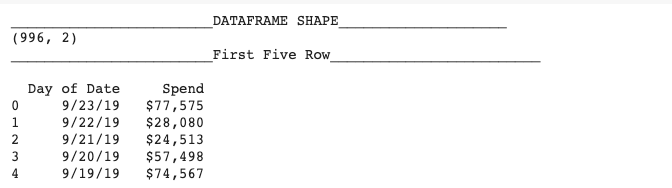``````df1 = data[['Date','Spend']].set_index('Date')
train = df1.iloc[:933,:]
test = df1.iloc[933:,:]
test.shape,train.shape``````

## 本文目录

1. 综述
• 时间序列分析常用统计模型
• 单变量时间序列数据建模的关键要素
1. ARIMA
• ACF 和 PACF
• SARIMA
• 案例：通过 SARIMA 预测广告支出
1. ETS
• ETS
• Holt-Winter 季节性预测算法
• 案例：通过 Holt-Winter 季节性预测算法预测广告支出
1. 算法对比
2. 结束语

## 综述

### 时间序列分析常用统计模型

• 单变量时间序列统计学模型，如：平均方法、平滑方法、有/无季节性条件的 ARIMA 模型。
• 多变量时间序列统计学模型，如：外生回归变量、VAR。
• 结构化时间序列模型，如：贝叶斯结构化时间序列模型、分层时间序列模型。

### 单变量时间序列统计学模型的关键要素

1. 变换，如：取对数、取平方等。
2. 平滑处理，如：移动平均等。
3. 差分。
4. 分解。
5. 多项式拟合，如：拟合回归。

## ARIMA

Autoregressive Integrated Moving Average model (ARIMA)，差分整合移动平均自回归模型。`ARIMA(p,d,q)`主要包含三项：

p:AR项，即自回归项(autoregression)，将时间序列下一阶段描述为前一阶段数据的线性映射。
d项，即积分项(integration)，时间序列的差分预处理步骤，使其满足平稳性要求
q:MA项，即移动平均项(moving average)，将时间序列下一阶段描述为前一阶段数据平均过程中的残留误差的线性映射。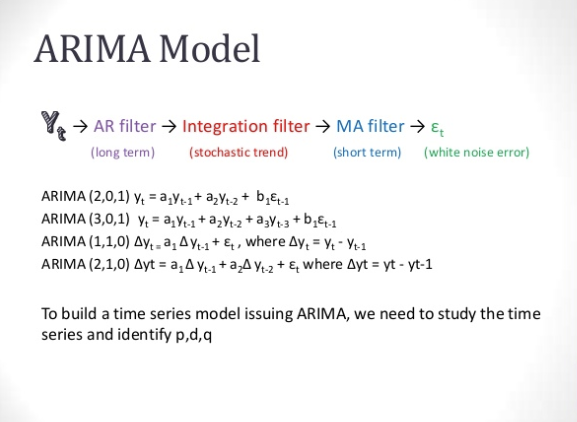### ACF 和 PACF 图

1. 通过 PACF 图可以确定 p
2. 通过 ACF 图可以确定 q

### 案例：通过 SARIMA 预测广告支出

``````from statsmodels.tsa.stattools import adfuller
df1=df.resample('D', how=np.mean)

def test_stationarity(timeseries):
rolmean = timeseries.rolling(window=30).mean()
rolstd = timeseries.rolling(window=30).std()

plt.figure(figsize=(14,5))
sns.despine(left=True)
orig = plt.plot(timeseries, color='blue',label='Original')
mean = plt.plot(rolmean, color='red', label='Rolling Mean')
std = plt.plot(rolstd, color='black', label = 'Rolling Std')

plt.legend(loc='best'); plt.title('Rolling Mean & Standard Deviation')
plt.show()

print ('<Results of Dickey-Fuller Test>')
dftest = adfuller(timeseries, autolag='AIC')
dfoutput = pd.Series(dftest[0:4],
index=['Test Statistic','p-value','#Lags Used','Number of Observations Used'])
for key,value in dftest.items():
dfoutput['Critical Value (%s)'%key] = value
print(dfoutput)
test_stationarity(df1.Spend.dropna())``````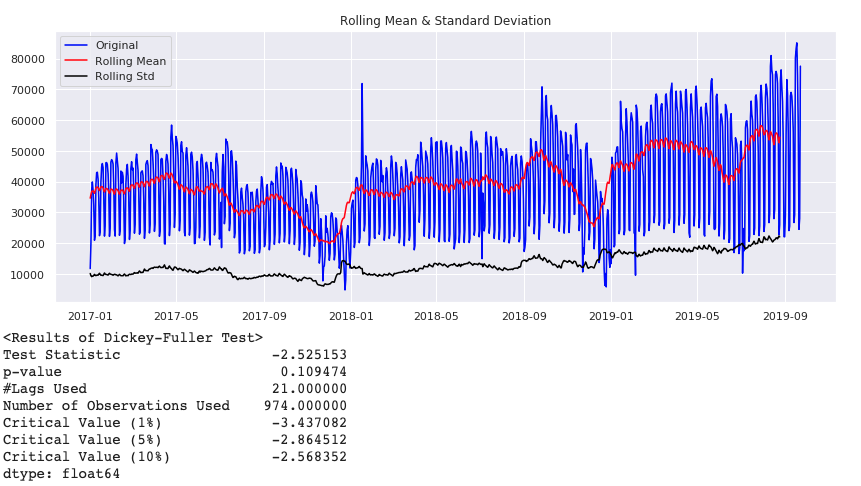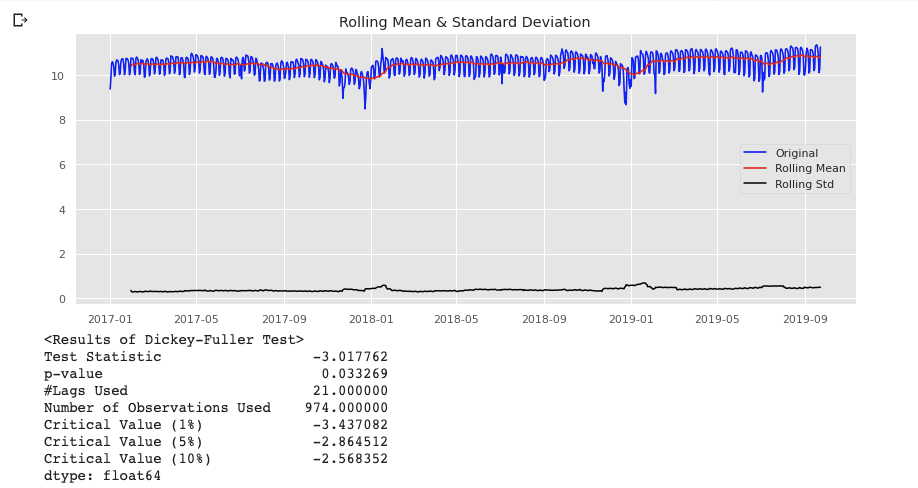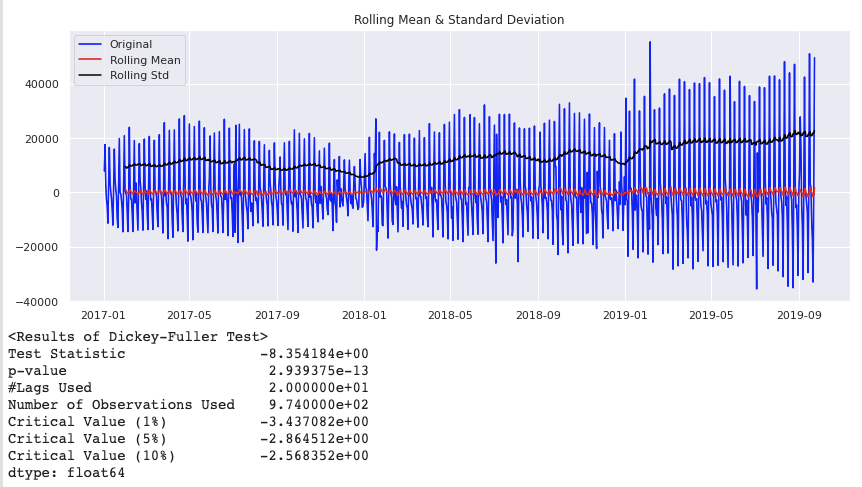``````import statsmodels.api as sm
fit1 = sm.tsa.statespace.SARIMAX(train.Spend, order=(7, 1, 2), seasonal_order=(0, 1, 2, 7)).fit(use_boxcox=True)
test['SARIMA'] = fit1.predict(start="2019-07-23", end="2019-09-23", dynamic=True)
plt.figure(figsize=(16, 8))
plt.plot(train['Spend'], label='Train')
plt.plot(test['Spend'], label='Test')
plt.plot(test['SARIMA'], label='SARIMA')
plt.legend(loc='best')
plt.show()``````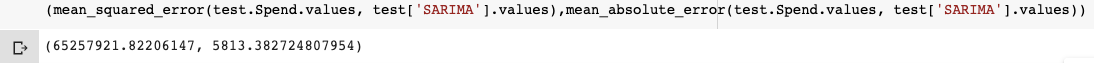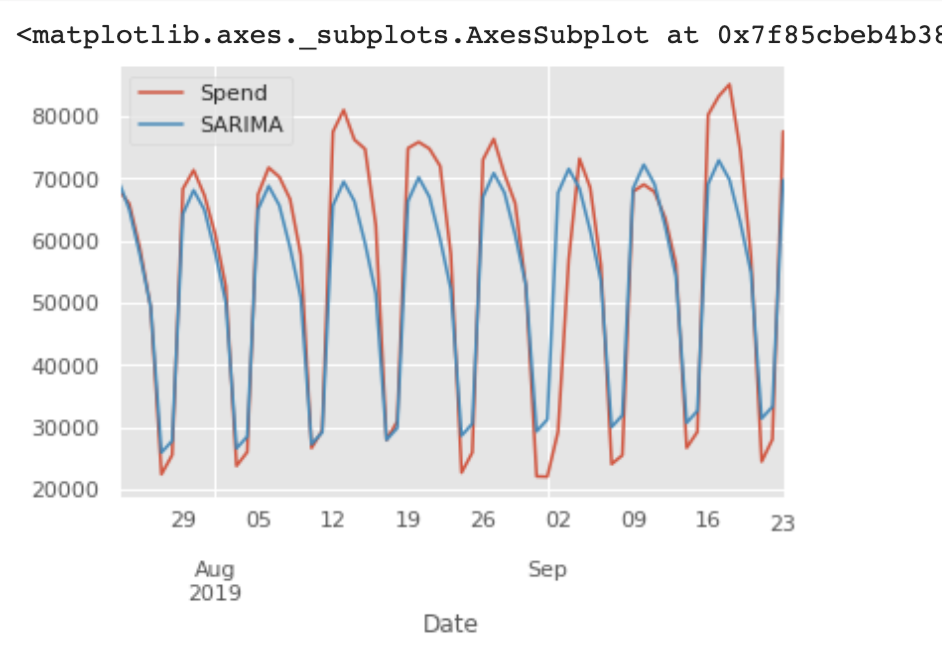## ETS

### ETS，Exponential Smoothing

• Linear：双指数平滑；
• Multiplicative：三指数平滑。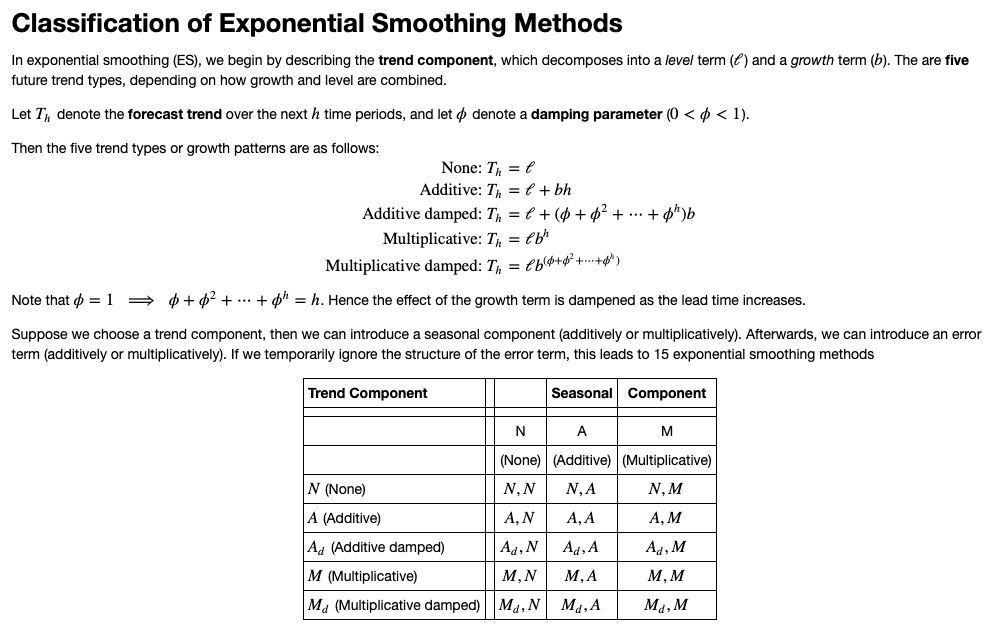### Holt-Winter 季节性预测算法

Holt-winter 季节性预测算法是一种三指数平滑方法。它包含三个主要部分：水平、趋势、季节性分量。

### 案例：通过 Holt-Winter 季节性预测算法预测广告支出

``````from statsmodels.tsa.api import ExponentialSmoothing
test['Holt_Winter'] = fit1.forecast(len(test))
plt.figure(figsize=(16,8))
plt.plot( train['Spend'], label='Train')
plt.plot(test['Spend'], label='Test')
plt.plot(test['Holt_Winter'], label='Holt_Winter')
plt.legend(loc='best')
plt.show()``````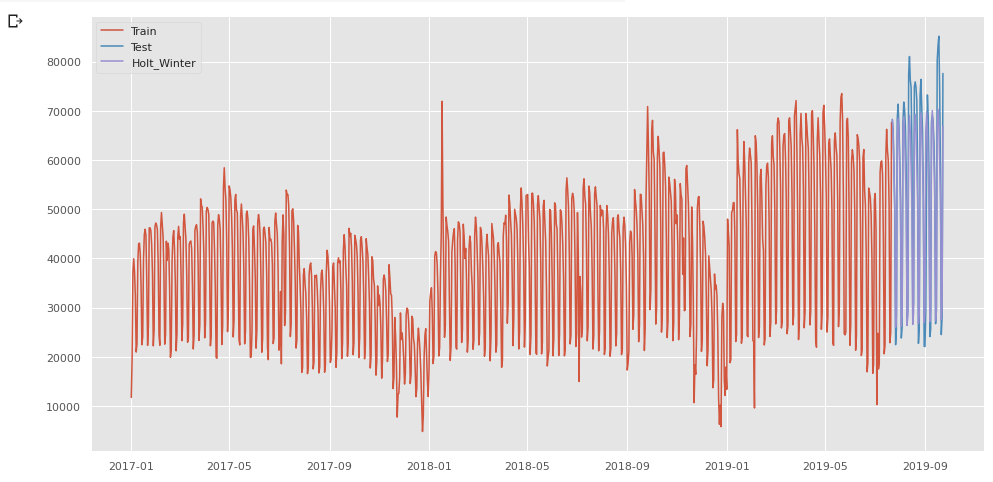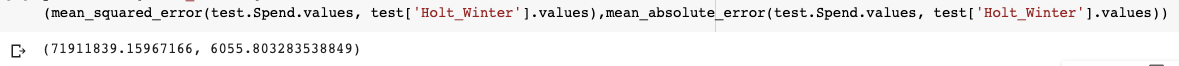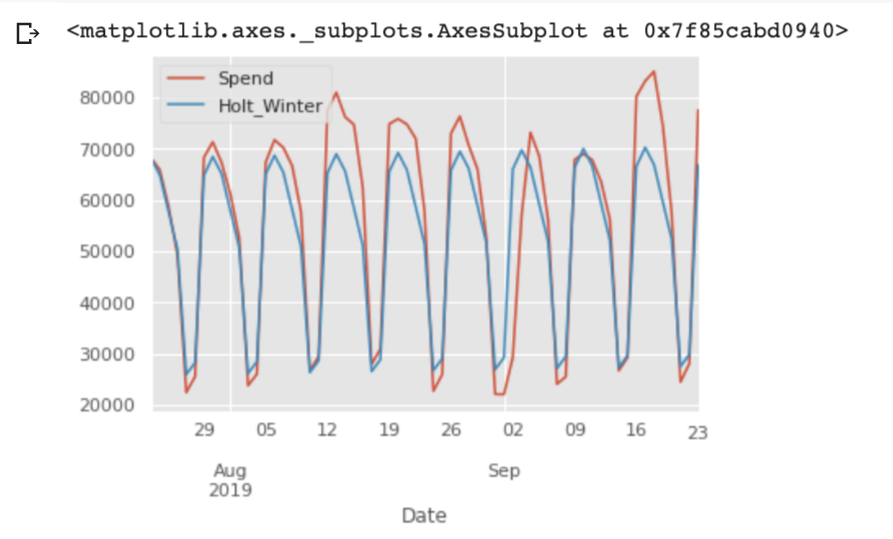## 算法对比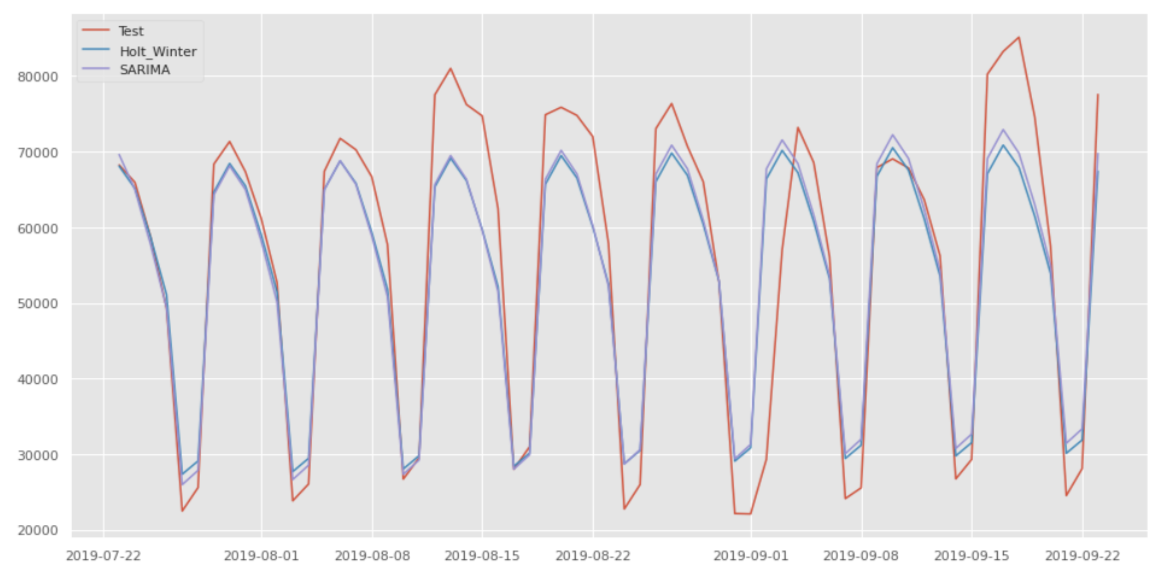## 结束语

###### 评论